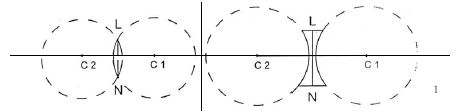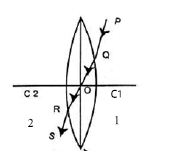Physics

# Few Definitions Related to Lens

Centre of curvature: Both surfaces of a lens are parts of different spheres. The centre of each sphere is called the centre of curvature of the respective surface. In figure, C1 and C2 are two centres of curvature of the lens LN. If one of the surfaces is plane instead of spherical then its centre of curvature will be at infinity.

Principal axis: Generally there are two spherical surfaces of a lens. The straight line passing through the two centres of curvature of the two spherical surfaces is called the principal axis. In figure, the straight line C1 C2 is the principal axis of the lens.Optical centre: The point on the principal axis inside a lens through which if a ray of light is passed then due to refraction if the ray emerges from the other surface being parallel to the incident ray then it is called optical centre.

In figure: 2, a ray of light PQ incidents on a surface of lens and refracts along the path QR. This ray emerges from the other surface along the path RS. The emergent ray RS and incident ray PQ are parallel to each other. The refracted ray QR intersects the principal axis C1 C2 at point 0 inside the lens. The point O is called the optical centre of the lens.